## Boolean-algebra

 Question 1

The simultaneous equations on the Boolean variables x, y, z and w,

```      x + y + z = 1
xy = 0
xz + w = 1
xy +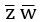= 0```

have the following solution for x, y, z and w, respectively.

 A 0 1 0 0 B 1 1 0 1 C 1 0 1 1 D 1 0 0 0
Digital-Logic-Design       Boolean-Algebra       GATE 2000
Question 1 Explanation:
Just put the values of each options in the equation and check it.
 Question 2

The Boolean function x'y' + xy + x'y is equivalent to

 A x' + y' B x + y C x + y' D x' + y
Digital-Logic-Design       Boolean-Algebra       GATE 2004
Question 2 Explanation:
x'y' + xy + x'y
= x'y' + x'y + xy
= x'(y'+y)+xy
= x'⋅1+xy
= x'+xy
= (x'+x)(x'+y)
= 1⋅(x'+y)
= x'+y
 Question 3

What values of A, B, C and D satisfy the following simultaneous Boolean equations?A A = 1, B = 0, C = 0, D = 1 B A = 1, B = 1, C = 0, D = 0 C A = 1, B = 0, C = 1, D = 1 D A = 1, B = 0, C = 0, D = 0
Digital-Logic-Design       Boolean-Algebra       GATE 1995
Question 3 Explanation:
For verification, just put up the values and check for AND, OR operations and their outputs.
 Question 4

What is the maximum number of different Boolean functions involving n Boolean variables?

 A n2 B 2n C 22n D 2n2
Digital-Logic-Design       Boolean-Algebra       GATE 2007
Question 4 Explanation:
Each “boolean” variable has two possible values i.e 0 and 1.
Number of variables= n
Number of input combinations is 2n.
Each “boolean” function has two possible outputs i.e 0 and 1.
Number of boolean functions possible is 22n.
Formula: The number of m-ary functions possible with n k-ary variables is mkn.
 Question 5

If P, Q, R are Boolean variables, thenSimplifies to

 ABC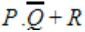D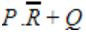Digital-Logic-Design       Boolean-Algebra       GATE 2008
Question 5 Explanation:Question 6

The number of min-terms after minimizing the following Boolean expression is ______.

`      [D′ + AB′ + A′C + AC′D + A′C′D]′    `
 A 1 B 2 C 3 D 4
Digital-Logic-Design       Boolean-Algebra       GATE 2015 [Set-2]       Video-Explanation
Question 6 Explanation:
Lets simplify it
[D' + AB' + A'C + AC'D + A'C'D]'
[D' + AB' + A'C + C'D (A + A')']' (since A+A' = 1)
[AB' + A'C + (D' + C') (D' + D)]' (since D' + D =1)
[AB' + A'C + D' + C']'
[AB' + (A' + C') (C + C') + D']'
[AB' + A' + C' + D']'
[(A + A') (A' + B') + C' + D']'
[A' + B' + C' + D']'
Apply de-morgan's law,
ABCD
 Question 7

Let, x1⊕x2⊕x3⊕x4 = 0 where x1, x2, x3, x4 are Boolean variables, and ⊕ is the XOR operator. Which one of the following must always be TRUE?

 A x1x2x3x4 = 0 B x1x3+x2 = 0 CD x1 + x2 + x3 + x4 = 0
Digital-Logic-Design       Boolean-Algebra       GATE 2016 [Set-2]       Video-Explanation
Question 7 Explanation:
Given expression is,
x1 ⊕ x2 ⊕ x3 ⊕ x4 = 0 -----(1)
A) x1x2x3 x4 = 0
Put x1 = 1, x2 = 1, x3 = 1, x4 = 1
The given equation will be zero, i.e.,
1 ⊕ 1 ⊕ 1 ⊕ 1 = 0
But,
x1x2x3 x4 ≠ 0
So, false.
B) x1x3 + x2 = 0
Put x1 = 1, x2 = 1, x3 = 0 , x4 = 0
The given equation will be zero, i.e.,
1 ⊕ 1 ⊕ 0 ⊕ 0 = 0
But,
x1x3 + x2 ≠ 0
So, false.
D) x1 + x2 + x3 + x4 = 0
Let x1=1, x2=1, x3=0, x4=0
The given equation will be zero, i.e.,
1 ⊕ 1 ⊕ 0 ⊕ 0 = 0
But,
x1 + x2 + x3 + x4 ≠ 0
So, false.
(i) True.
 Question 8

Consider the Boolean operator with the following properties:Then x#y is equivalent to

 AB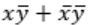C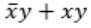DDigital-Logic-Design       Boolean-Algebra       GATE 2016 [Set-1]       Video-Explanation
Question 8 Explanation: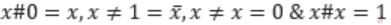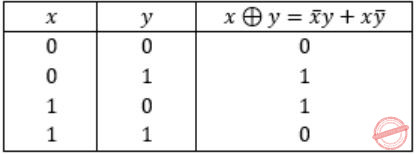Ex-OR satisfies all the properties. Hence,Question 9

Consider the following Boolean expression for F:

`   F(P, Q, R, S) = PQ + P'QR + P'QR'S`

The minimal sum-of-products form of F is

 ABC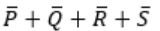DDigital-Logic-Design       Boolean-Algebra       GATE 2014 [Set-1]       Video-Explanation
Question 9 Explanation:
PQ + P’QR + P’QR’S
= Q(P+P’R) + P’QR’S
= Q(P+R) + P’QR’S
= QP + QR + P’QR’S
= QP + Q(R + P’R’S)
= QP + Q( R + P’S)
= QP + QR + QP’S
= Q(P+P’S) + QR
= Q(P+S)+ QR
= QP + QS + QR
 Question 10

The minterm expansion of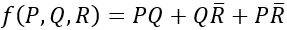is

 A m2+m4+m6+m7 B m0+m1+m3+m5 C m0+m1+m6+m7 D m2+m3+m4+m5
Digital-Logic-Design       Boolean-Algebra       GATE 2010
Question 10 Explanation:
Convert PQ + QR' + PR' into canonical form
= PQR + PQR' + PQR' + P'QR' + PQR' + PQ'R'
= PQR + PQR' + P'QR' + PQ'R'
= m7 + m6 + m2 + m4
 Question 11

The simplified SOP (sum of product) form of the boolean expressionABCDDigital-Logic-Design       Boolean-Algebra       GATE 2011
Question 11 Explanation:Question 12

The truth tablerepresents the Boolean function

 A X B X + Y C X ⊕ Y D Y
Digital-Logic-Design       Boolean-Algebra       GATE 2012
Question 12 Explanation:
f(X,Y) = XY’ + XY = X(Y’ + Y) = X
There are 12 questions to complete.

Register Now